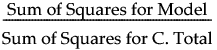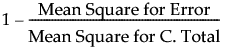Publication date: 04/12/2021

## Summary of Fit Report

#### RSquare

Using quantities from the corresponding analysis of variance table, the RSquare for any continuous response fit is calculated as follows:#### RSquare Adj

The RSquare Adj is a ratio of mean squares instead of sums of squares and is calculated as follows:The mean square for Error is in the Analysis of Variance report (Figure 5.12). You can compute the mean square for C. Total as the Sum of Squares for C. Total divided by its respective degrees of freedom.

Want more information? Have questions? Get answers in the JMP User Community (community.jmp.com).